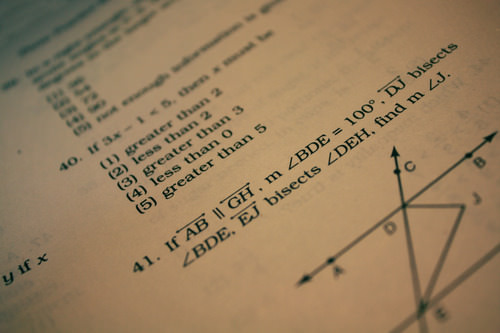# 3.6: Inequalities with Multiplication and Division

•• Contributed by CK12
• CK12

## Solve Inequalities Using MultiplicationFigure $$\PageIndex{1}$$

Therese, a grade 12 student, has been accepted by a prestigious university into their Advanced Math Studies Program. The university is hosting a Math competition for its incoming students to win a $5,000 entrance scholarship. The winner will be the contestant who correctly answers the most questions within the allotted time. Her coach reads her this inequality to solve. “If one-third Melvin’s age six years from now is greater than 14, what will his age have to be?” Therese needs to figure out a solution set for Melvin’s age? What inequality must she solve to answer the question? In this concept, you will learn to solve inequalities using multiplication. ### Multiplying Inequalities An inequality is a mathematical statement that can be identified by the presence of an inequality symbol. These symbols are > , ≥, < and ≤. Besides using the addition and subtraction properties of inequality, the multiplication property of inequality can also be used to solve inequalities. The Multiplication Property of Inequality states that if each side of an inequality is multiplied by the same positive number, the sense (inequality symbol) of the inequality remains unchanged. The following rules of the multiplication property can be used to solve an inequality: • If then a>b and c>0, then a×c>b×c • If then a≥b and c>0, then a×c≥b×c • If then a<b and c>0, then a×c<b×c • If then a≤b and c>0, then a×c≤b×c However, if both sides of the inequality are multiplied by the same negative number then the sense (inequality symbol) of the inequality changes and the symbol must be reversed. The following rules of the multiplication property can be used to solve an inequality. • If then a>b and c<0, then a×c<b×c • If then a≥b and c<0, then a×c≤b×c • If then a<b and c<0, then a×c>b×c • If then a≤b and c<0, then a×c≥b×c Both sides of an inequality are multiplied by the same number when the quantity is expressed as a fraction and the inverse operation for division has to be applied to solve for the variable. Let’s apply the multiplication property of inequality to solve an inequality. Solve the following inequality for the set of real numbers. (x/5)−(4/5)≥3 First, isolate the variable by adding 4/5 to both sides of the inequality. (x/5)−(4/5)≥3 (x/5)−(4/5)+(4/5)≥3+(4/5) Next, simplify both sides of the inequality. (x/5)−(4/5)+(4/5)≥3+(4/5) (x/5)≥3(5/5)+(4/5) (x/5)≥(15/5)+(4/5) (x/5)≥(19/5) Then, multiply both sides of the inequality by 5 to solve for the variable ‘x.’ This is where the multiplication property of equality is applied to solving the inequality. (x/5)≥(19/5) 5(x/5)≥5(19/5) x≥19 The solution is {x|x≥19,x∈R}. ### Examples Example 3.6.1 Earlier, you were given a problem about Therese and the$5,000 entrance scholarship. She needs to solve an inequality to figure out a solution set for Melvin’s age.

Solution

First, write a verbal model to represent the information given.

Verbal Model: one third Melvin’s age six years from now will be greater than 14.

Next, name the variable Let ‘a’ represent Melvin’s age.

Next, translate the verbal model to an inequality.

1/3(a+6)>14

Next, apply the distributive property to clear the parenthesis.

1/3(a+6)>14

1/3(a)+1/3(6)>14

(a/3)+2>14

Next, isolate the variable by subtracting 2 from both sides of the inequality.

(a/3)+2>14

(a/3)+2−2>14−2

Next, simplify both sides of the inequality.

(a/3)+2−2>14−2

(a/3)>12

Then, multiply both sides of the inequality by 3 to solve for ‘a.’

(a/3)>12

(3/1)(a/3)>3(12)

a>36

Melvin’s age must be greater than 36 and belong to the set of Natural numbers.

The solution set is {a|a>36,a∈N}

Example 3.6.2

Solve the following inequality for the set of whole numbers.

(m−9)/4≤2

Solution

First, multiply both sides of the inequality by 4.

(m−9)/4≤2

4((m−9)/4)≤4(2)

Next, simplify both sides of the inequality.

(4/1)((m−9)/4)≤4(2)

m−9≤8

Next, isolate the variable by adding 9 to both sides of the inequality.

m−9≤8

m−9+9≤8+9

Then, simplify both sides of the inequality.

m−9+9≤8+9

m≤17

The solution set is {m|m≤17,m∈W}.

Example 3.6.3

Insert the correct inequality sign in each of the following by using the rules of the multiplication property of inequality.

i) If m<n then 4⋅m___4⋅n

ii) if x≥y then −12⋅x___12⋅y

iii) If 8<10 then (−2)(8)___(−2)(10)

Solution

Part i): Both sides of the inequality are multiplied by a positive number. Therefore, the sense of the inequality remains unchanged.

Part ii): Both sides of the inequality are multiplied by a negative number. Therefore, the sense of the inequality changes. The inequality symbol must be reversed from ≥ to ≤.

Part iii): Both sides of the inequality are multiplied by a negative number. Therefore, the sense of the inequality changes. The inequality symbol must be reversed from <to >.

Example 3.6.4

Solve the following inequality for the set of real numbers.

(1/2)x−3≥7

Solution

First, isolate the variable by adding 3 to both sides of the inequality.

(1/2)x−3≥7

(1/2)x−3+3≥7+3

Next, simplify both sides of the inequality.

(1/2)x−3+3≥7+3

(1/2)x≥10

Next, multiply both sides of the inequality by 2 to solve for the variable ‘x.’

(1/2)x≥10

2((1/2)x)≥2(10)

Then simplify both sides of the inequality.

(2/1)((1/2)x)≥2(10)

x≥20

The solution set is {x|x≥20,x∈R}.

### Review

Solve each inequality by applying the multiplication property of inequality.

1. a/3<10
2. x/2>20
3. b/4<25
4. x/5>100
5. c/2≤20
6. x/7>6
7. y/9≤2
8. y/11≥5
9. x/2<3
10. y/8≥−7
11. x/3<−2
12. y/4≤−3
13. x/2≥−11
14. a/13≥−3
15. x/22≥−5

### Vocabulary

Term Definition
inequality An inequality is a mathematical statement that relates expressions that are not necessarily equal by using an inequality symbol. The inequality symbols are <, >, ≤, ≥ and ≠.
Multiplication Property of Inequality The multiplication property of inequality states that when an inequality is multiplied by a negative number, the sense of the inequality changes and the sign is reversed.